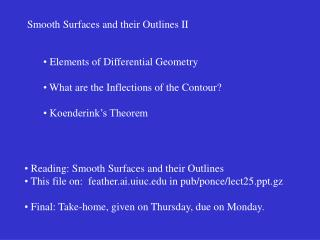# Smooth Surfaces and their Outlines II - PowerPoint PPT PresentationDownload PresentationSmooth Surfaces and their Outlines II

Smooth Surfaces and their Outlines II
Download Presentation## Smooth Surfaces and their Outlines II

- - - - - - - - - - - - - - - - - - - - - - - - - - - E N D - - - - - - - - - - - - - - - - - - - - - - - - - - -
##### Presentation Transcript

1. Smooth Surfaces and their Outlines II • Elements of Differential Geometry • What are the Inflections of the Contour? • Koenderink’s Theorem • Reading: Smooth Surfaces and their Outlines • This file on: feather.ai.uiuc.edu in pub/ponce/lect25.ppt.gz • Final: Take-home, given on Thursday, due on Monday.

2. Smooth Shapes and their Outlines Can we say anything about a 3D shape from the shape of its contour?

3. What are the contour stable features?? folds cusps T-junctions Shadows are like silhouettes..

4. Differential geometry: geometry in the small The normal to a curve is perpendicular to the tangent line. A tangent is the limit of a sequence of secants.

5. What can happen to a curve in the vicinity of a point? (a) Regular point; (b) inflection; (c) cusp of the first kind; (d) cusp of the second kind.

6. The Gauss Map It maps points on a curve onto points on the unit circle. The direction of traversal of the Gaussian image reverts at inflections: it fold there.

7. The curvature C • C is the center of curvature; • R = CP is the radius of curvature; • k = lim dq/ds = 1/R is the curvature.

8. Twisted curves are more complicated animals..

9. A smooth surface, its tangent plane and its normal.

10. Normal sections and normal curvatures Principal curvatures: minimum value k maximum value k Gaussian curvature: K = k k 1 1 2 2

11. The local shape of a smooth surface Elliptic point Hyperbolic point K > 0 K < 0 K = 0 Parabolic point

12. The Gauss map The Gauss map folds at parabolic points.

13. Smooth Shapes and their Outlines Can we say anything about a 3D shape from the shape of its contour?

14. Theorem [Koenderink, 1984]: the inflections of the silhouette are the projections of parabolic points.

15. Koenderink’s Theorem (1984) K = kk r c Note: k > 0. r Corollary: K and k have the same sign! c

16. From Horn’s Robot Vision